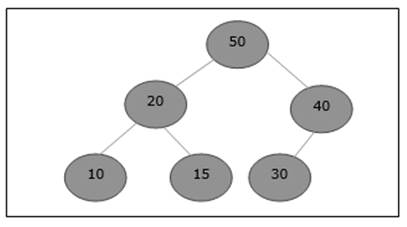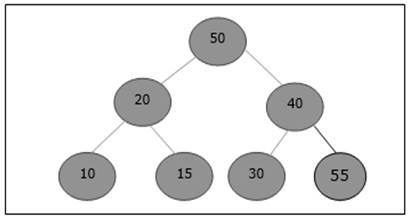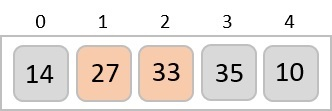• Design and Analysis of Algorithms
• Home

# Design and Analysis Insert Method

Advertisements

To insert an element in a heap, the new element is initially appended to the end of the heap as the last element of the array.

After inserting this element, heap property may be violated, hence the heap property is repaired by comparing the added element with its parent and moving the added element up a level, swapping positions with the parent. This process is called percolation up.

The comparison is repeated until the parent is larger than or equal to the percolating element.

```Algorithm: Max-Heap-Insert (numbers[], key)
heapsize = heapsize + 1
numbers[heapsize] = -∞
i = heapsize
numbers[i] = key
while i > 1 and numbers[Parent(numbers[], i)] < numbers[i]
exchange(numbers[i], numbers[Parent(numbers[], i)])
i = Parent (numbers[], i)
```

## Analysis

Initially, an element is being added at the end of the array. If it violates the heap property, the element is exchanged with its parent. The height of the tree is log n. Maximum log n number of operations needs to be performed.

Hence, the complexity of this function is O(log n).

## Example

Let us consider a max-heap, as shown below, where a new element 5 needs to be added.Initially, 55 will be added at the end of this array.After insertion, it violates the heap property. Hence, the element needs to swap with its parent. After swap, the heap looks like the following.Again, the element violates the property of heap. Hence, it is swapped with its parent.Now, we have to stop.

Advertisements